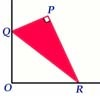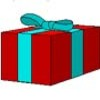#### You may also likeA circle is inscribed in an equilateral triangle. Smaller circles touch it and the sides of the triangle, the process continuing indefinitely. What is the sum of the areas of all the circles?### Set Square

A triangle PQR, right angled at P, slides on a horizontal floor with Q and R in contact with perpendicular walls. What is the locus of P?A box of size a cm by b cm by c cm is to be wrapped with a square piece of wrapping paper. Without cutting the paper what is the smallest square this can be?

# Ford Circles

##### Age 16 to 18Challenge Level
The GeoGebra applet below shows the construction of a sequence of Ford Circles. Ford Circles have centre $\left(\frac{p}{q},\frac1{2q^2}\right)$ and radius $\frac1{2q^2}$, where $\frac{p}{q}$ is a fraction in its simplest form (that is, $p$ and $q$ are coprime integers).

In the interactivity below, you can move the sliders to choose values for $a, b, c$ and $d$. The circles have centres $\left(\frac{a}{c},\frac1{2c^2}\right)$ and $\left(\frac{b}{d},\frac1{2d^2}\right)$, and radii $\frac1{2c^2}$ and $\frac1{2d^2}$.

When the two circles touch, they are coloured in blue.
Explore the interactivity and find some values of $a, b, c$ and $d$ that generate circles that touch each other.

In the problem Farey Neighbours, you are invited to explore the value of $ad-bc$ for two adjacent fractions $\frac bd$ and $\frac ac$ from any Farey Sequence.

Explore the value of $ad-bc$ for the touching circles that you have found.
What do you notice?

Can you prove that for any touching circles in the interactivity above, $|ad-bc|=1$?
Can you prove that, given two such circles which touch the $x$ axis at $\frac bd$ and $\frac ac$, the circle with centre $\left(\frac{a+b}{c+d},\frac1{2(c+d)^2}\right)$ and radius $\frac1{2(c+d)^2}$ is tangent to both circles?